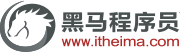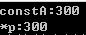# C/C++培训专家：C/C++中const的区别

Let’s begin!!!!

c语言全局const会被存储到只读数据段。c++中全局const当声明extern或者对变量取地址时，编译器会分配存储地址，变量存储在只读数据段。两个都受到了只读数据段的保护，不可修改。

const int constA = 10;

int main(){

int* p = (int*)&constA;

*p = 200;

}

c语言中局部const存储在堆栈区，只是不能通过变量直接修改const只读变量的值，但是可以跳过编译器的检查，通过指针间接修改const值。

const int constA = 10;

int* p = (int*)&constA;

*p = 300;

printf("constA:%d\n",constA);

printf("*p:%d\n", *p);c语言中，通过指针间接赋值修改了constA的值。

c++中对于局部的const变量要区别对待：

1.对于基础数据类型，也就是const int a = 10这种，编译器会把它放到符号表中，不分配内存，当对其取地址时，会分配内存。

const int constA = 10;

int* p = (int*)&constA;

*p = 300;

cout << "constA:" << constA << endl;

cout << "*p:" << *p << endl;constA在符号表中，当我们对constA取地址，这个时候为constA分配了新的空间，*p操作的是分配的空间，而constA是从符号表获得的值。

1.对于基础数据类型，如果用一个变量初始化const变量，如果const int a = b,那么也是会给a分配内存。

int b = 10;

const int constA = b;

int* p = (int*)&constA;

*p = 300;

cout << "constA:" << constA << endl;

cout << "*p:" << *p << endl;constA 分配了内存，所以我们可以修改constA内存中的值。

1.对于自定数据类型，比如类对象，那么也会分配内存。

const Person person; //未初始化age

//person.age = 50; //不可修改

Person* pPerson = (Person*)&person;

//指针间接修改

pPerson->age = 100;

cout << "pPerson->age:" << pPerson->age << endl;

pPerson->age = 200;

cout << "pPerson->age:" << pPerson->age << endl;c中const默认为外部连接，c++中const默认为内部连接.当c语言两个文件中都有const int a的时候，编译器会报重定义的错误。而在c++中，则不会，因为c++中的const默认是内部连接的。如果想让c++中的const具有外部连接，必须显示声明为: extern const int a = 10;

const由c++采用，并加进标准c中，尽管他们很不一样。在c中，编译器对待const如同对待变量一样，只不过带有一个特殊的标记，意思是”你不能改变我”。在c++中定义const时，编译器为它创建空间，所以如果在两个不同文件定义多个同名的const，链接器将发生链接错误。简而言之,const在c++中用的更好。